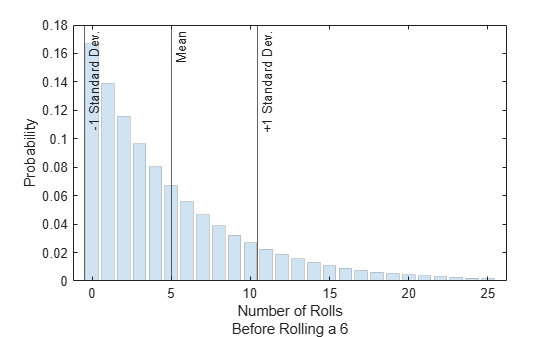geostat

Geometric mean and variance

Description

example

[m,v] = geostat(p) returns the mean m and variance v of a geometric distribution with the corresponding probability parameter in p. For more information, see Geometric Distribution Mean and Variance.

Examples

collapse all

Roll a fair die repeatedly until you successfully get a 6. The associated geometric distribution models the number of times you roll the die before the result is a 6. Determine the mean and variance of the distribution, and visualize the results.

Because the die is fair, the probability of successfully rolling a 6 in any given trial is p = 1/6. Compute the mean and variance of the geometric distribution.

p = 1/6;
[m,v] = geostat(p)
m = 5.0000

v = 30.0000

Notice that the mean m is $\left(1-p\right)/p$ and the variance v is $\left(1-p\right)/{p}^{2}$.

m2 = (1-p)/p
m2 = 5.0000

v2 = (1-p)/p^2
v2 = 30.0000

Evaluate the probability density function (pdf), or probability mass function (pmf), at the points x = 0,1,2,...,25.

rng("default") % For reproducibility
x = 0:25;
y = geopdf(x,p);

Plot the pdf values. Indicate the mean, one standard deviation below the mean, and one standard deviation above the mean.

bar(x,y,"FaceAlpha",0.2,"EdgeAlpha",0.2);
xline([m-sqrt(v) m m+sqrt(v)],"-", ...
["-1 Standard Dev.","Mean","+1 Standard Dev."])
xlabel(["Number of Rolls","Before Rolling a 6"])
ylabel("Probability")Create a probability vector that contains three different parameter values.

• The first parameter corresponds to a geometric distribution that models the number of times you toss a coin before the result is heads.

• The second parameter corresponds to a geometric distribution that models the number of times you roll a four-sided die before the result is a 4.

• The third parameter corresponds to a geometric distribution that models the number of times you roll a six-sided die before the result is a 6.

p = [1/2 1/4 1/6];

Compute the mean and variance of each geometric distribution.

[m,v] = geostat(p)
m = 1×3

1.0000    3.0000    5.0000

v = 1×3

2.0000   12.0000   30.0000

The returned values indicate that, for example, the mean of a geometric distribution with probability parameter p = 1/4 is 3, and the variance of the distribution is 12.

Input Arguments

collapse all

Probability of success in a single trial, specified as a scalar or an array of scalars in the range [0,1]. To compute the means and variances of multiple distributions, specify the distribution parameters p using an array of scalar values.

Example: 0.5

Example: [1/2 1/3]

Data Types: single | double

Output Arguments

collapse all

Mean of the geometric distribution, returned as a numeric scalar or an array of numeric scalars. m is the same size as p, and each element in m is the mean of the geometric distribution specified by the corresponding element in p.

Variance of the geometric distribution, returned as a numeric scalar or an array of numeric scalars. v is the same size as p, and each element in v is the variance of the geometric distribution specified by the corresponding element in p.

collapse all

Geometric Distribution Mean and Variance

The geometric distribution is a one-parameter family of curves that models the number of failures before a success occurs in a series of independent trials. Each trial results in either success or failure, and the probability of success in any individual trial is constant. For example, if you toss a coin, the geometric distribution models the number of tails observed before the result is heads. The geometric distribution is discrete, existing only on the nonnegative integers.

The mean of the geometric distribution is $\text{mean}=\frac{1-p}{p}\text{\hspace{0.17em}},$ and the variance of the geometric distribution is $\mathrm{var}=\frac{1-p}{{p}^{2}}\text{\hspace{0.17em}},$ where p is the probability of success.

 Abramowitz, M., and I. A. Stegun. Handbook of Mathematical Functions. New York: Dover, 1964.

 Evans, M., N. Hastings, and B. Peacock. Statistical Distributions. 2nd ed., Hoboken, NJ: John Wiley & Sons, Inc., 1993.